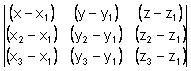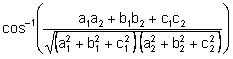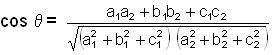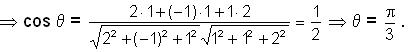×#### Thank you for registering.

One of our academic counsellors will contact you within 1 working day.

Click to Chat

1800-1023-196

+91-120-4616500

CART 0

• 0

MY CART (5)

Use Coupon: CART20 and get 20% off on all online Study Material

ITEM
DETAILS
MRP
DISCOUNT
FINAL PRICE
Total Price: Rs.

There are no items in this cart.
Continue Shopping
```
I want vector equations of a plane and line please send me the formulas

```
one year ago

```							Dear student  Equation of Plane in Different Forms		General equation of a plane is ax + by + cz + d = 0			Equation of the plane in Normal form is lx + my + nz = p where p is the length of the normal from the origin to the plane and (l, m, n) be the direction cosines of the normal.			The equation to the plane passing through P(x1, y1, z1) and having direction ratios			(a, b, c) for its normal is a(x – x1) + b(y – y1) + c (z – z1) = 0			The equation of the plane passing through three non-collinear points (x1, y1, z1),			(x2, y2, z2) and (x3, y3 , z3) is= 0			The equation of the plane whose intercepts are a, b, c on the x, y, z axes respectively is x/a + y/b + z/c = 1 (a b c ≠ 0)			Equation of YZ plane is x = 0, equation of plane parallel to YZ plane is x = d.	Equation of ZX plane is y = 0, equation of plane parallel to ZX plane is y = d.Equation of XY plane is z = 0, equation of plane parallel to XY plane is z = d.	Four points namely A (x1, y1, z1), B (x2, y2, z2), C (x3, y3, z3) and D (x4, y4, z4) will be coplanar if one point lies on the plane passing through other three points.CXmhaU -8: Find the equation to the plane passing through the point (2, -1, 3) which is the foot of the perpendicular drawn from the origin to the plane.        hb: The direction ratios of the normal to the plane are 2, -1, 3.The equation of required plane is 2(x –2) –1 (y + 1) + 3 (z –3) = 0=> 2x – y + 3z –14 = 0Angle between the PlanesAngle between the planes is defined as angle between normals of the planes drawn from any point to the planes.Angle between the planes a1x + b1y + c1z + d1 = 0 and a2x + b2y + c2z + d2 = 0 isNote:		If a1a2 +b1b2 +c1c2 = 0, then the planes are perpendicular to each other.			If a1/a2 = b1/b2 = c1/c2 then the planes are parallel to each other.	CXmhaU -9: Find angle between the planes 2x – y + z = 11 and x + y + 2z = 3.hb:CXmhaU -10: Find the equation of the plane passing through (2, 3, –4), (1, –1, 3) and parallel to x-axis.hb: The equation of the plane passing through (2, 3, –4) isa(x – 2) + b(y – 3) + c(z + 4) = 0                              ……(1)since (1, –1, 3) lie on it, we havea + 4b – 7c = 0                                                          ……(2)since required plane is parallel to x-axis i.e. perpendicular to YZ plane i.e.1.a + 0.b + 0.c = 0 Þ a = 0 Þ 4b – 7c = 0 => b/7 = c/4∴ Equation of required plane is 7y + 4z = 5.Perpendicular Distance:The length of the perpendicular from the point P(x1, y1, z1) to the plane ax + by + cz + d = 0 is|ax1 + by1 + cz1 + d / √a2 + b2 + c2|.Family of Planes:Equation of plane passing through the line of intersection of two planes u = 0 and v = 0 is u + λv = 0.Intersection of a Line and Plane:If equation of a plane is ax + by + cz + d = 0, then direction cosines of normal to this plane are a, b, c. So angle between normal to the plane and a straight line having direction cosines l, m ,n is given by cos θ = al + bm + cn / √a2 + b2 + c2.Then angle between the plane and the straight line is π/2 – θ.	Plane and straight line will be parallel if al + bm + cn = 0	Plane and straight line will be perpendicular if a/l = b/m = c/n.
```
one year ago
Think You Can Provide A Better Answer ?

## Other Related Questions on Vectors

View all Questions »### Course Features

• 731 Video Lectures
• Revision Notes
• Previous Year Papers
• Mind Map
• Study Planner
• NCERT Solutions
• Discussion Forum
• Test paper with Video Solution

### Course Features

• 19 Video Lectures
• Revision Notes
• Test paper with Video Solution
• Mind Map
• Study Planner
• NCERT Solutions
• Discussion Forum
• Previous Year Exam Questions